# Chapter 6 More about Factors

This was part of a impromptu session learning about factors.

``````# load libraries
library(tidyverse)
library(janitor)
library(forcats)

sheet=1,
na="NA")``````

## 6.1 Making a factor variable out of disease

We’re adding a fourth value, `BRCA` to our levels here.

``````smoke_complete2 <- smoke_complete %>%
mutate(disease_factor =
factor(disease,
levels = c("LUSC", "CESC", "BLCA", "BRCA")
)
) ``````

## 6.2 Using the character variable

``````ggplot(smoke_complete2) +
aes(x=disease, y=cigarettes_per_day) +
geom_boxplot()``````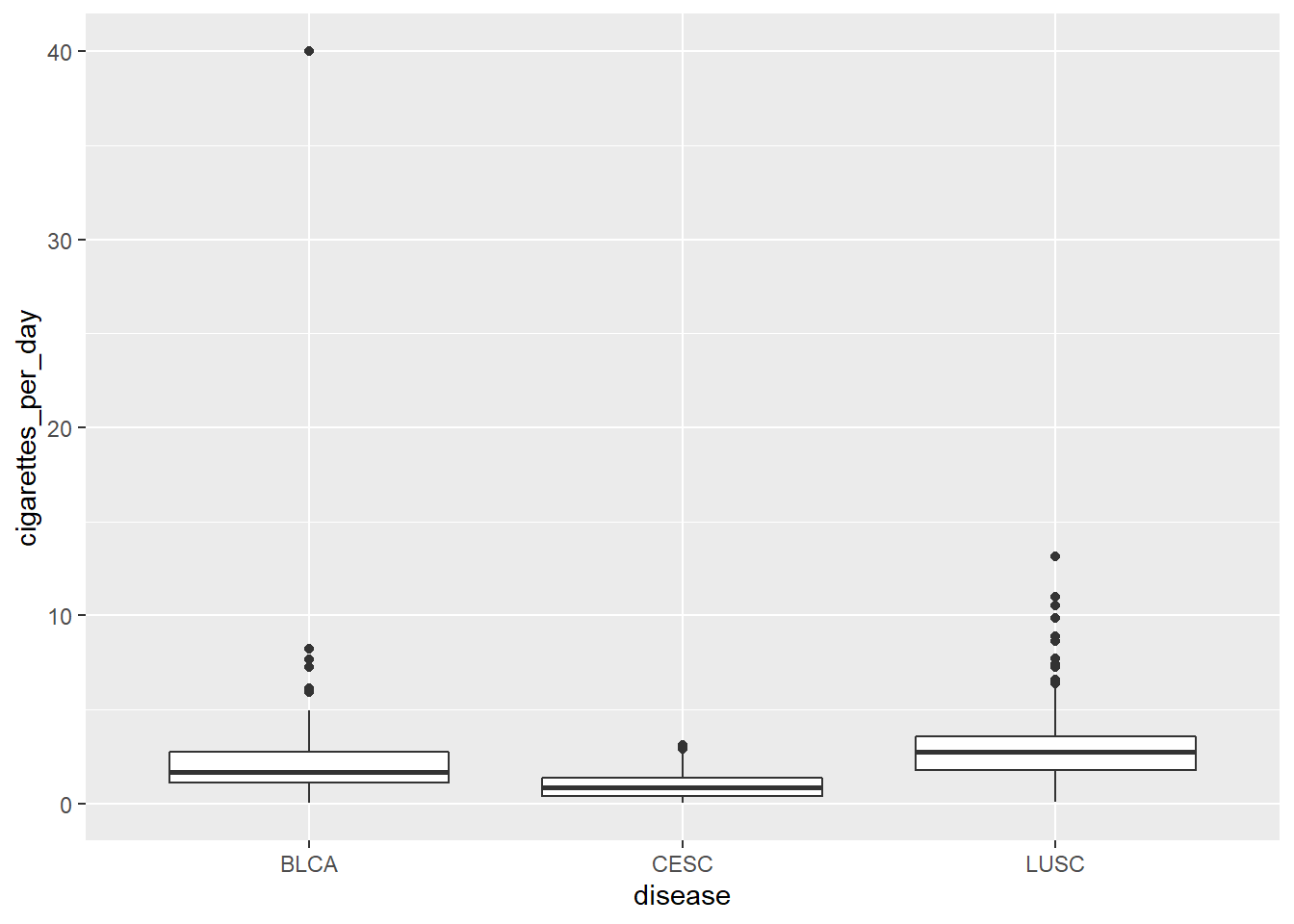## 6.3 Compare to the factor variable

``````ggplot(smoke_complete2) + aes(x=disease_factor, y=cigarettes_per_day) +
geom_boxplot()``````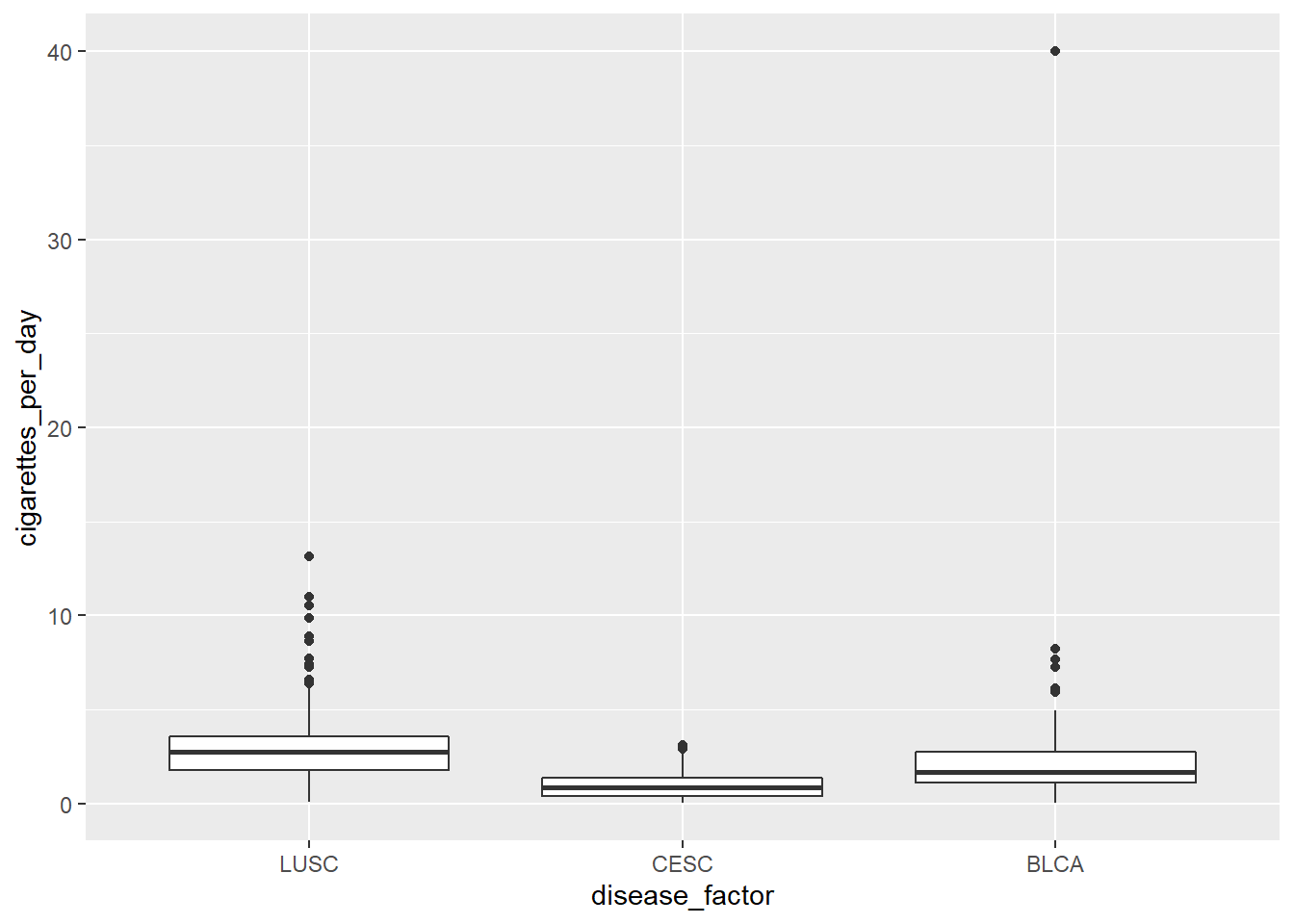## 6.4 Another thing about factors

Factor `levels` also specify the permissible values.

In this example, `LUSC` and `BRCA` are the permissible values. We pass a character vector into them, and you can see those values (`BLCA`, `CESC`) are recoded as NAs

``````character_vector <- c("LUSC", "LUSC", "BRCA", "BLCA", "BRCA", "CESC", "CESC")

factor_vector <- factor(character_vector, levels=c("LUSC", "BRCA"))

factor_vector``````
``````##  LUSC LUSC BRCA <NA> BRCA <NA> <NA>
## Levels: LUSC BRCA``````

## 6.5`fct_rev()` - reversing the order of a factor

Very useful when using factors on the y-axis, because the default ordering is first value at the bottom, rather than first value at the top.

``````library(forcats)

#fct_rev()

smoke_complete3 <- smoke_complete2 %>%
mutate(disease_rev = fct_rev(disease_factor))

#show original factor
ggplot(smoke_complete3) + aes(y=disease_factor, x=cigarettes_per_day) +
geom_boxplot()``````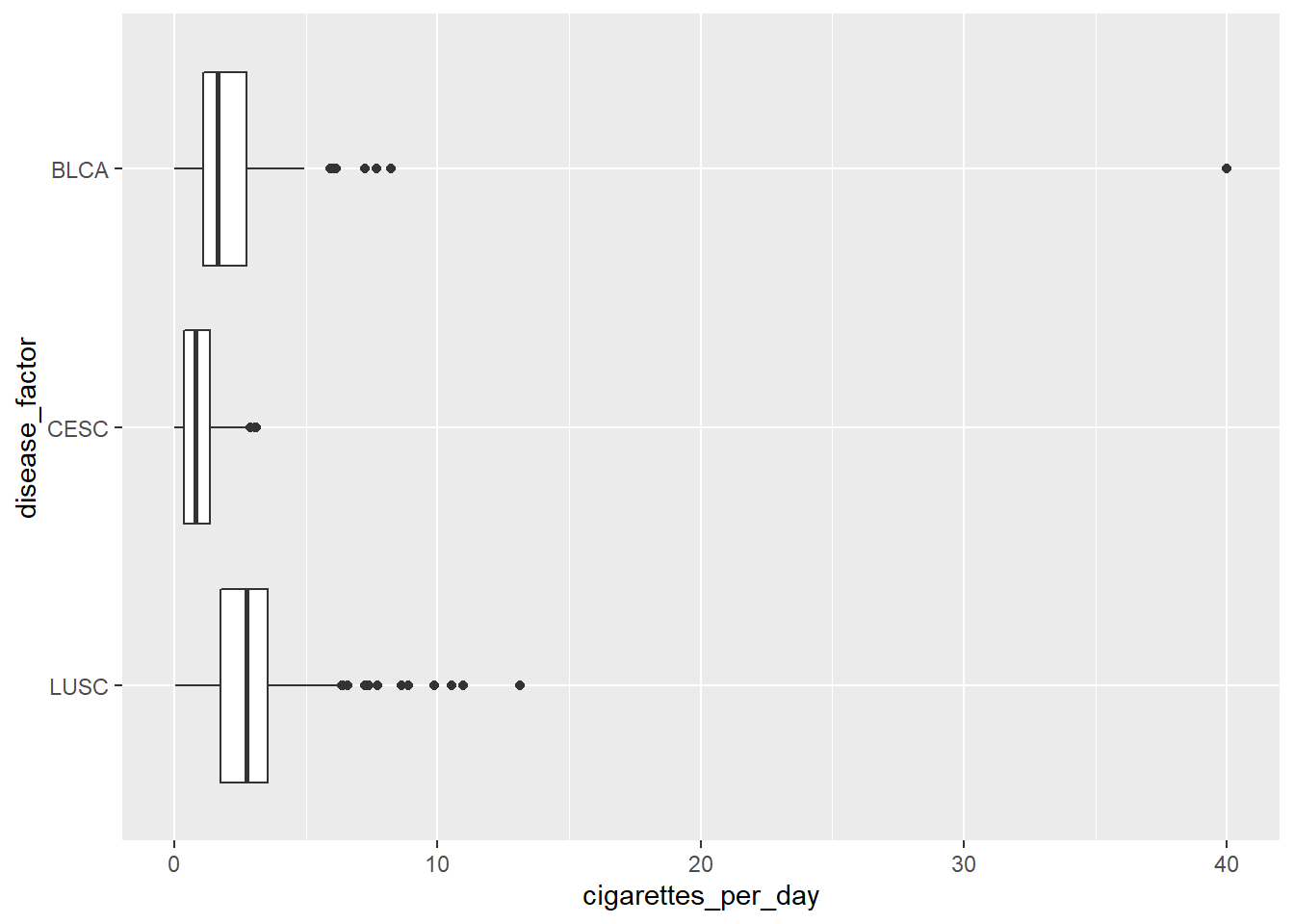``````#show factor with reversed order
ggplot(smoke_complete3) + aes(y=disease_rev, x=cigarettes_per_day) +
geom_boxplot()``````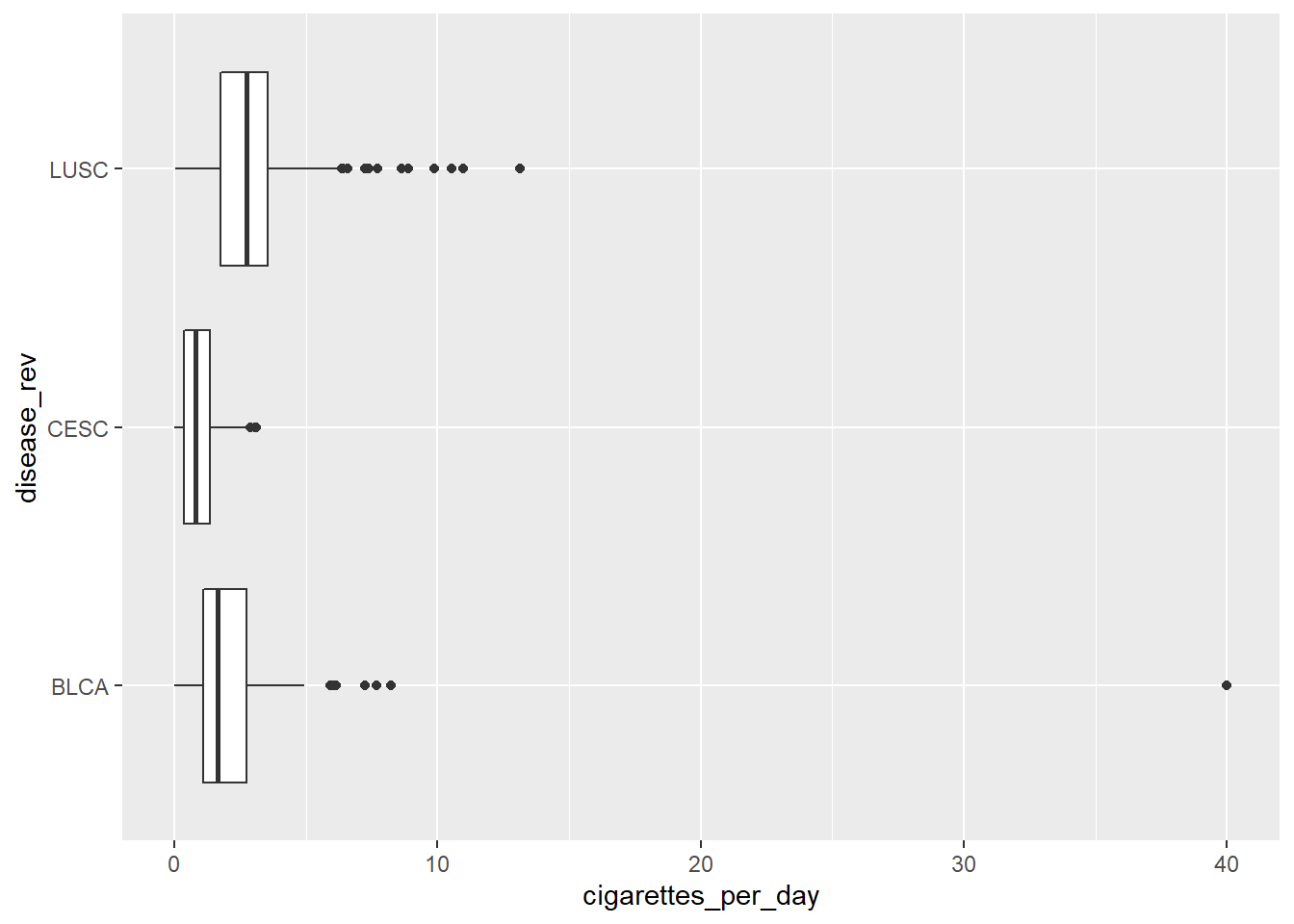## 6.6`fct_reorder()`

`fct_reorder()` lets you reorder factors by another `numeric` variable.

``````library(forcats)

#fct_rev()

smoke_complete3 <- smoke_complete2 %>%
mutate(disease_reorder = fct_reorder(disease_factor, cigarettes_per_day))

ggplot(smoke_complete3) + aes(y=disease_reorder, x=cigarettes_per_day) +
geom_jitter()``````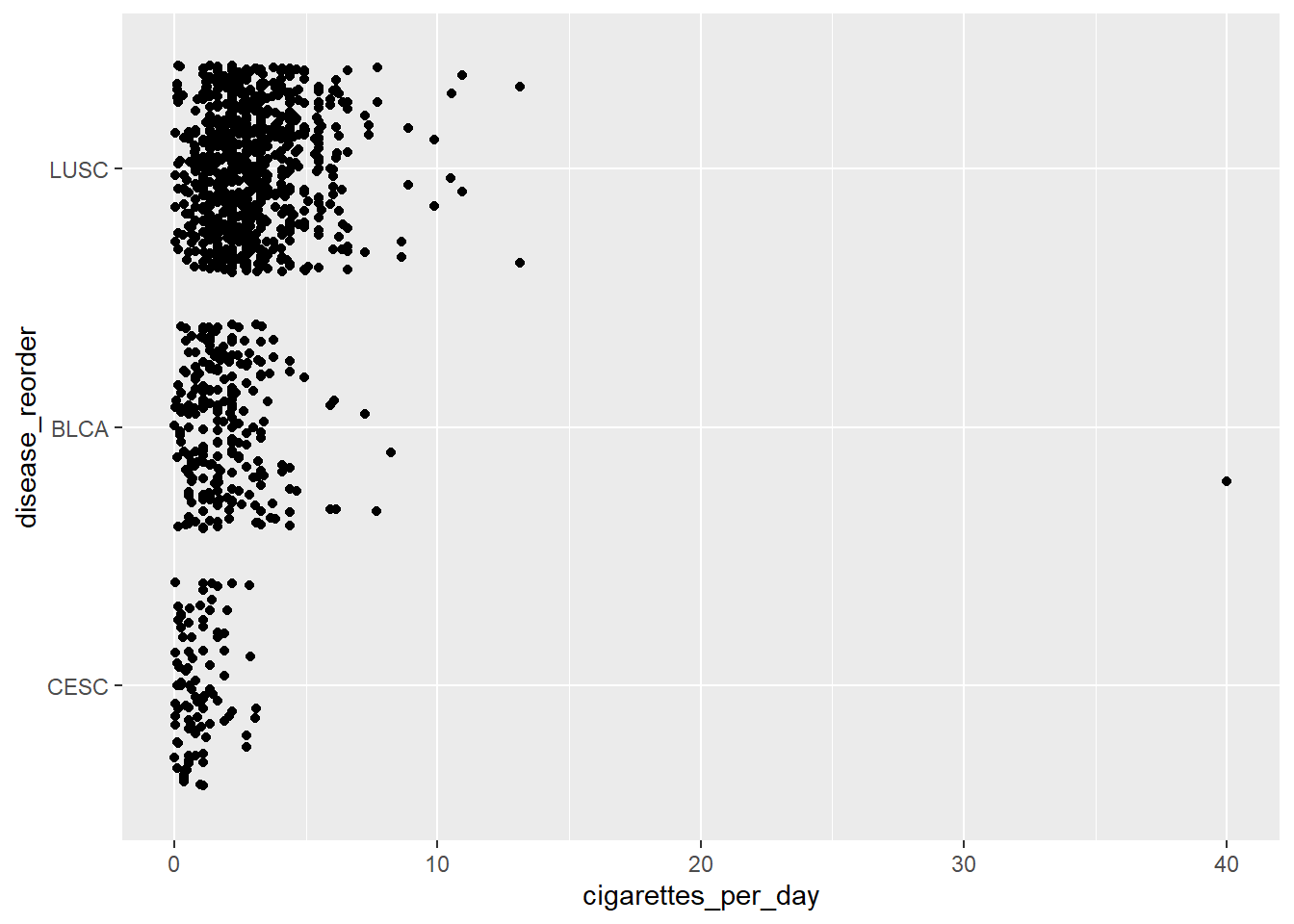## 6.7`fct_collapse`

`fct_collapse()` lets you collapse multiple categories into one category.

``````smoke_complete3 %>%
mutate(disease_collapse = fct_collapse(
disease_factor,
other = c("BLCA", "CESC"),
LUSC = c("LUSC")
)) %>%
tabyl(disease_collapse)``````
``````##  disease_collapse   n   percent
##              LUSC 836 0.7256944
##             other 316 0.2743056
##              BRCA   0 0.0000000``````

## 6.8 Other really useful `forcats` functions

`fct_recode()` - lets you recode values manually.

`fct_other()` - lets you define what categories are in an `other` variable.